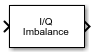Main Content

# I/Q Imbalance

Apply I/Q imbalances to complex signal

• Library:
• Communications Toolbox / RF Impairments

•## Description

The I/Q Imbalance block applies in-phase and quadrature imbalances to a complex signal. This block applies an amplitude imbalance, a phase imbalance, and a DC offset to the in-phase and quadrature signal components. For more information, see I/Q Imbalance Implementation and Algorithms.

## Ports

### Input

expand all

Complex signal, specified as a scalar or vector.

Data Types: `double` | `single`
Complex Number Support: Yes

### Output

expand all

Output signal, returned as a scalar or vector. This output is the same dimension and data type as the input signal.

## Parameters

expand all

I/Q amplitude imbalance in decibels of signal power, specified as a scalar. For more information, see Algorithms.

I/Q amplitude imbalance in degrees, specified as a scalar.

In-phase component DC offset, specified as a scalar.

Quadrature component DC offset, specified as a scalar.

## Block Characteristics

 Data Types `double` | `single` Multidimensional Signals `no` Variable-Size Signals `no`

expand all

## Algorithms

The I/Q amplitude imbalance, I/Q phase imbalance, and DC offset impairments are described sequentially in the section.

1. For an I/Q amplitude imbalance, Ia, the impairment is applied to the input signal, xr+ jxi and y AmplitudeImbalance is an intermediate output.

y AmplitudeImbalance $\triangleq$ y rAmplitudeImbalance + jyiAmplitudeImbalance

y AmplitudeImbalance = $\left({10}^{\left(0.5{I}_{\text{a}}/20\right)}{x}_{\text{r}}\right)+j\left({10}^{\left(-0.5{I}_{\text{a}}/20\right)}{x}_{\text{i}}\right)$

2. For an I/Q phase imbalance, Ip, the impairment is applied to y AmplitudeImbalance and yPhaseImbalance is an intermediate output.

yPhaseImbalance $\triangleq$ yrPhaseImbalance + jyiPhaseImbalance

yPhaseImbalance =$\left({e}^{\left(j\left(-0.5\pi \frac{{I}_{p}}{180}\right)\right)}{y}_{{\text{r}}_{\text{AmplitudeImbalance}}}\right)+\left({e}^{\left(j\left(\frac{\pi }{2}+0.5\pi \frac{{I}_{\text{p}}}{180}\right)\right)}{y}_{{\text{r}}_{\text{AmplitudeImbalance}}}\right)$

3. For DC offsets, IDC and QDC, the impairment is applied to y PhaseImbalance and y is the final output.

y = (yrPhaseImbalance + IDC) + j(yiPhaseImbalance + QDC)

Variables for these calculations are defined in this list.

• I a is the I/Q amplitude imbalance.

• Ip is the I/Q phase imbalance.

• IDC is the in-phase DC offset.

• QDC is the quadrature DC offset.

• x is the complex input signal and is given by xr + jxi.

• xr and xi are the real and imaginary parts, respectively, of x.

• y is the complex output signal and is given by yr + jyi.

• yr and yi are the real and imaginary parts, respectively, of y.

## Extended Capabilities

### C/C++ Code GenerationGenerate C and C++ code using Simulink® Coder™.

Introduced before R2006a

## Support

#### Bridging Wireless Communications Design and Testing with MATLAB

Download white paper